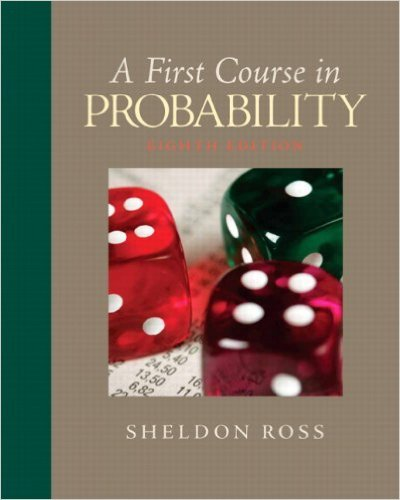# Given 20 people, what is the probability that,among the 12## Problem 48 Chapter 2

First Course in Probability | 8th Edition

• 2901 Step-by-step solutions solved by professors and subject experts
• Get 24/7 help from StudySoup virtual teaching assistantsFirst Course in Probability | 8th Edition

4 5 0 354 Reviews
29
0
Problem 48

Given 20 people, what is the probability that,among the 12 months in the year, there are 4months containing exactly 2 birthdays and 4 containingexactly 3 birthdays?

Step-by-Step Solution:
Step 1 of 3

X, Y Bivariate Random Variables f(u1, 2 ) is density of (X,Y) ∫ ( ) density of X ∫ ( ) density of Y X, Y are independent if f(u1, 2 = f1(u1 2 (u2) If X1, ,2…….X are nndependent Var(X 1 ……..X ) = n + …….. We want to produce to specification “m” “m” = length of a part, content of juice can etc We want to check at the...

Step 2 of 3

Step 3 of 3

##### ISBN: 9780136033134

Unlock Textbook Solution

Given 20 people, what is the probability that,among the 12

×
Get Full Access to First Course In Probability - 8 Edition - Chapter 2 - Problem 48

Get Full Access to First Course In Probability - 8 Edition - Chapter 2 - Problem 48

I don't want to reset my password

Need help? Contact support

Need an Account? Is not associated with an account
We're here to help